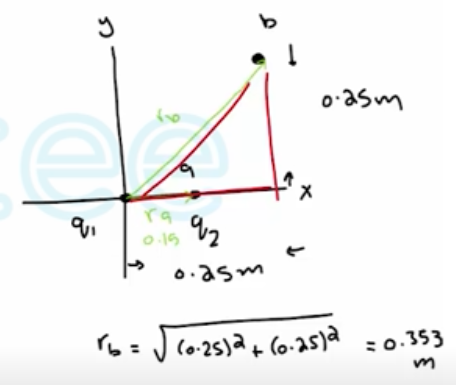Need Help?

Subscribe to Physics 2

###### \${selected_topic_name}
• Notes

*$W=F \cdot d=q_0 E d=U$*

*$U=\frac{1}{4 \pi \varepsilon_{0}} \frac{q q_{0}}{r}$*

*$U=\frac{1}{4 \pi \varepsilon_0} \frac{e^{2}}{r}$*

$\left(e=1.60 * 10^{-14} c\right)$

A point charge $q_{1}=+2.40 \mu \mathrm{C}$ is held stationary at the
origin. A second point charge $q_{2}=-4.30 \mu \mathrm{C}$ moves from
the point $x=0.150 \mathrm{m}, \quad y=0$ to the point $x=0.250 \mathrm{m}$ ,
$y=0.250 \mathrm{m} .$ How much work is done by the electric force on $q_{2} ?$$W_{a \rightarrow b}=U_{a}-U_{b}$

$U_{a}=\frac{1}{4 \pi \varepsilon_{0}} \frac{q_{1} q_{2}}{r_{a}}$

$=9 * 10^{9} * \frac{2.4 * 10^{-6} *-4 \cdot 3 * 10^{-6}}{0.15}$

$=-0.6184 \mathrm{J}$

$U_{b}=\frac{1}{4 \pi \varepsilon_{0}} \frac{q_{1} q_{2}}{r_{b}}$

$=9 * 10^{9} * \quad \frac{2 \cdot 4 * 10^{-6} *-4 \cdot 3*10^{-6}}{0.3536}$

$=-0.26235J$

Three equal $1.20-\mu \mathrm{C}$ point charges are placed at the
corners of an equilateral triangle whose sides are 0.500 m long.
What is the potential energy of the system? (Take as zero the
potential energy of the three charges when they are infinitely
far apart.)

$U=U_{12}+U_{13}+U_{23}$

$=U_{12}=U_{13}=U_{23}$

$U=\frac{3 k q^{2}}{0.5} =\frac{3 * K *\left(1.2 * 10^{-6}\right)^{2}}{0.5}$

$=0.078 J$